# Law Of Conservation Of Matter

The law of conservation of matter means that matter is not created or destroyed but changes . This means that the mass before the chemical reaction is equal to the mass after the reaction.

This law of chemistry is also known as law of conservation of the mass or law of Lavoisier. In a chemical reaction, substances (reactants) rearrange their atoms to give rise to other substances (products).

## Definition of the law of conservation of matter

“The mass of a closed or isolated system remains unchanged regardless of the transformation that occurs within it.”

closed system is one where there is no interaction with the environment. In this sense, within a closed system substances do not enter or leave, but energy can be released. The isolated system , like the closed, there is no input or output of matter, but there is no transfer of energy.

## Applications of the law of conservation of matter

The law of conservation of matter was a key milestone in the progress of chemistry. Thanks to this it can be established that substances do not disappear or are created out of nothing in a chemical reaction.

### Balance of the chemical equation

The chemical equation shows the participants of the chemical reaction. We speak of a balanced chemical equation when the amount of atoms on the side of the reactants is equal to the side of the products. For example: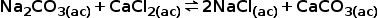In this reaction between sodium carbonate and calcium chloride, sodium chloride and calcium carbonate are produced. The atoms of the reactants and the products are shown in the table;

Element Reactants products
Sodium (Na) two two
Carbon (C) one one
Calcium (Ca) one one
Oxygen (O) 3 3
Chlorine (Cl) two two

As we can see, the atoms of the reactants are equal to the atoms of the products.

### Predict the mass of reactants and products

The conservation of the mass says that the total mass of the reactants must be equal to the total mass of the products. For example, in a closed container we have 160 g of oxygen and 68 g of NH 3 ammonia , which is equal to 228 g of reactants. The chemical reaction that occurs is as follows: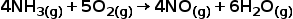We will have at the end of the reaction the quantity of 228 g of nitric oxide (NO) and water (H 2 ) as products.

## Discovery of the law of conservation of matter

It was the Russian scientist Mikhail Lomonosov (1711-1765) who first demonstrated the conservation of matter in 1756. He did so by showing how lead plates did not change weight while burning in a sealed container (without contact with air). ). Unfortunately, this finding went unnoticed for many years.

Lavoisier, in one of his experiments, boiled water for 101 days in a special bottle, which condensed the water vapor and returned it to the bottle. In this way no substance was lost in the course of the experiment. When they compared the weight of the container before and after the boiling period, they were the same. Lavoisier in this way established that the mass was not created or destroyed but was transformed from one substance to another .

## Easy experiment to demonstrate the law of conservation of matter

For this experiment we need:

• baking soda (4gr)
• vinegar (10 ml)
• a scale for weighing
• a jar with a wide mouth and a lid
• test tubes

### First activity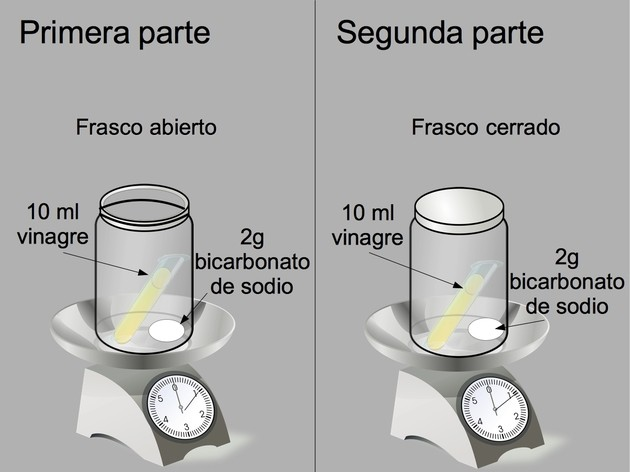Easy experiment to demonstrate the law of conservation of matter.

On a scale, place a wide-mouthed bottle with 2 grams of baking soda and inside this a test tube with 5 ml of vinegar. We weigh and write down the value. Then we spill the contents of the test tube into the bottle. When the bubbles finish, we write down the new value of the weight.

### Second activity

On the same scale, we placed a wide-mouthed bottle with 2 grams of sodium bicarbonate and inside this a test tube with 5 ml of vinegar and closed the bottle. We weigh and write down the value. Then we take the bottle and pour the contents of the test tube inside. When the bubbles finish, we write down the new value of the weight.

Caution : due to the formation of gas (carbon dioxide) the pressure inside the closed bottle increases. One must be very careful when opening it, doing it slowly so that the gas is released little by little.

### conclusion

Vinegar is diluted acetic acid. Acetic acid reacts with sodium bicarbonate to form sodium acetate and carbon dioxide, according to the following equation;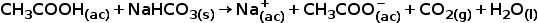In the first activity, when the vinegar reacts with the sodium bicarbonate, the carbon dioxide is released into the atmosphere since the bottle is open. In this case the weight decreases.

See also Chemical reactions .

## Law of conservation of matter and energy

Albert Einstein established in his theory of special relativity:

“… the mass of a body is a measure of its energy content …”

The total energy content of a body can be calculated by multiplying its body with the speed of light squared: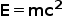According to this, there is no effective distinction between mass and energy: energy and mass are equivalent. In fact, the law of conservation of matter derives from the conservation of energy. If a body loses energy it also loses mass; really this is not loss but a transfer to the environment.

### Example

In the combustion of 342 g of common sugar (sucrose):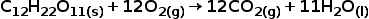5,635 kJ of energy is released. This amount of energy equals 6.269 x 10 -11 kg, an extremely small amount of mass difficult to measure with current techniques.

## Exercises to apply the law of conservation of matter

### Exercise 1

When heating 10 g of calcium carbonate (CaCO 3 ), 4.4 g of carbon dioxide (CO 2) and 5,6 g of calcium oxide (CaO) are produced. Is the law of conservation of mass fulfilled in this experiment?

Solution : We have to calculate the mass of the reactants and the mass of the products:The mass of the products is equal to 5.6g + 4.4g = 10g.

We then have, as expected, that 10 grams of CaCO 3 are transformed into 10 grams of CaO and CO 2 products .

### Exercise 2

Ammonia NH 3 is produced by the reaction of nitrogen and hydrogen: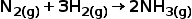What is the maximum amount of ammonia mass that the mixture of 1000 g N 2and 500 g of H 2 can produce ? What is the mass of the initial material that did not react?

Then 28 g of N 2 react with 6 g of H 2 , 1000 g of N 2 will react with:Then, 1000 g of N 2 plus 214.20 g of H 2 produce the maximum amount of 1214.20 g of ammonia. This means that the material that did not react was hydrogen: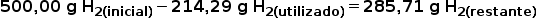### Exercise 3

In the following reaction: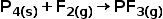What is the mass of fluorine (F 2 ) needed to produce 120 g of PF 3 ? The atomic weight of phosphorus P is 31 g / mol and that of fluorine is 19 g / mol.

Solution : first we have to verify that the equation is balanced. There are 4 P atoms in the reactants and 1 P atom in the products. If we place a 6 in front of the F 2 and a 4 in front of PF 3 , we balance the equation in the following way: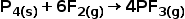Element Reactants products
Phosphorus (P) 4 4
Fluorine (F) 12 12

We now know that 1 mol of P 4 plus 6 mol of F 2 produce 4 mol of PF 3 , therefore, 124 g of P 4 plus 228 g of F 2 produce 352 g of PF 3 . We want to know how much fluorine is needed to produce 120 g of phosphorus trifluoride.Answer : 77.7 g of F 2 is needed to produce 120 g of PF 3 .

Close
• ### Atomic Model Of Bohr

Close